• +91 9971497814
• info@interviewmaterial.com

# Rd Chapter 9- Triangle and its Angles Ex-9.2 Interview Questions Answers

### Related Subjects

Question 1 : The exterior angles, obtained on producing the base of a triangle both way are 104° and 136°. Find all the angles of the triangle.

Answer 1 : In the given problem, the exterior angles obtained on producing the base of a triangle both ways are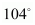and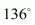. So, let us draw ΔABC and extend the base BC, such that: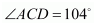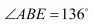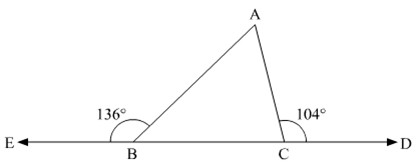Here, we need to find all the three angles of the triangle.
Now, since BCD is a straight line, using the property, “angles forming a linear pair are supplementary”, we get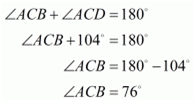Similarly, EBC is a straight line, so we get,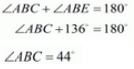Further, using angle sum property in ΔABC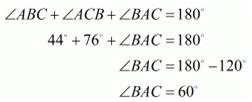Therefore,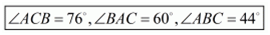Question 2 : In a Δ ABC, the internal bisectors of ∠B and ∠C meet at P and the external bisectors of ∠B and ∠C meet at Q, Prove that ∠BPC + ∠BQC = 180°.

In the given problem, BP and CP arethe internal bisectors of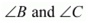respectively. Also, BQ and CQ arethe external bisectors ofrespectively. Here, we need toprove: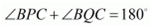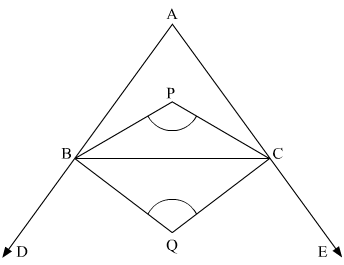We know that if the bisectors of angles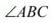and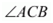of ΔABC meetat a point then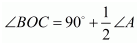Thus, in ΔABC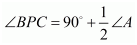……(1)

Also, using the theorem, “if the sides AB and AC of a ΔABC are produced, and theexternal bisectors of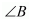and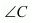meet at O, then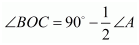Thus, ΔABC

BQC=90°−12A        ......(2)BQC=90°-12A        ......2

Adding (1) and (2), we get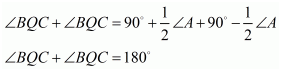Thus,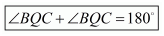Hence proved.

Question 3 : In the given figure, the sides BC, CA and AB of a Δ ABC have been produced to D, E and F respectively. If ∠ACD = 105° and ∠EAF = 45°, find all the angles of the Δ ABC.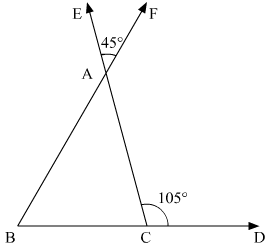In the given ΔABC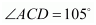and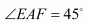. We need to find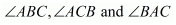.Here,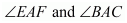are vertically oppositeangles. So, using the property, “vertically opposite angles are equal”, we get,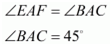Further, BCD is a straight line. So, usinglinear pair property, we get,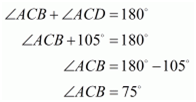Now, in ΔABC, using “the angle sum property”, we get,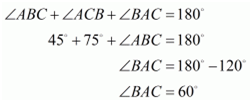Therefore,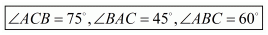Question 4 : Compute the value of x in each of the following figures:

(i)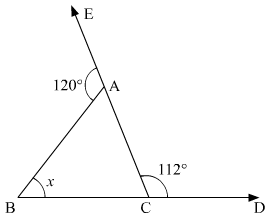(ii)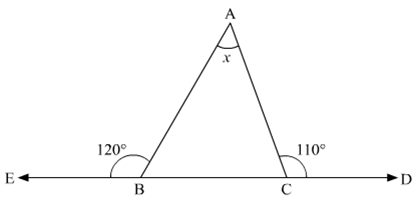(iii)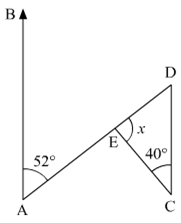(iv)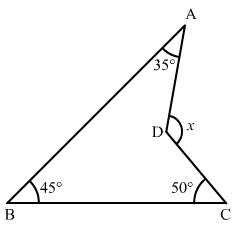In the given problem, we need to find the value of x

(i) In the given ΔABC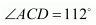and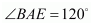Now, BCD is a straight line. So, using theproperty, “the angles forming a linear pair are supplementary”, we get,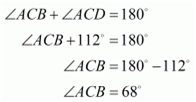Similarly, EAC is a straight line. So, we get,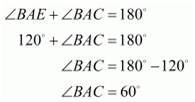Further, using the angle sum property of a triangle,

In ΔABC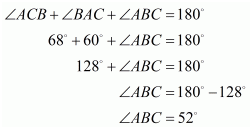Therefore,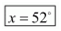(ii) In the given ΔABC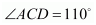and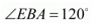Here, BCD is a straight line. So, using theproperty, “the angles forming a linear pair are supplementary” we get,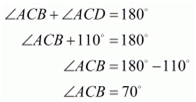Similarly, EBC is a straight line. So, we get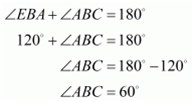Further, using the angle sum property of a triangle,

In ΔABC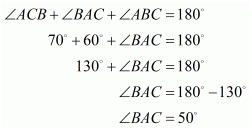Therefore,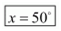(iii) In the given figure,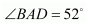and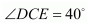Here,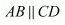and AD is thetransversal, so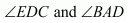form a pair of alternate interiorangles. Therefore, using the property, “alternate interior angles are equal”,we get,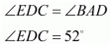Further, applying angle sum property of the triangle

In ΔDEC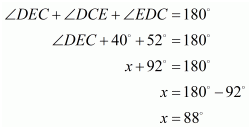Therefore,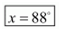(iv) In the given figure,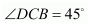,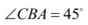and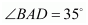Here, we will produce AD to meet BC at E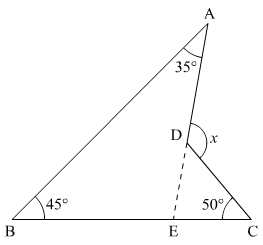Now, using angle sum property of the triangle

In ΔAEB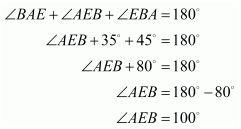Further, BEC is a straight line. So, using theproperty, “the angles forming a linear pair are supplementary”, we get,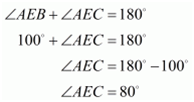Also, using the property, “an exterior angle of a triangle isequal to the sum of its two opposite interior angles”

In ΔDECx is its exterior angle

Thus,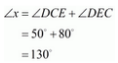Therefore,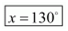.

Question 5 :

In the given figure, AB divides DAC in the ratio 1 : 3 and AB = DB. Determinethe value of x.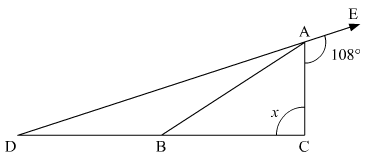In the given figure,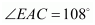and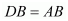Since,and angles opposite to equal sidesare equal. We get,

Also, EAD is a straight line. So, using theproperty, “the angles forming a linear pair are supplementary”, we get,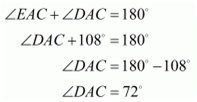Further, it is given  AB divides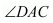in the ratio 1 : 3.

So, let

DAB=yBAC=3yDAB=y, BAC=3y

Thus,

y+3y=DAC4y=72°y=72°4y=18°y+3y=DAC4y=72°y=72°4y=18°

Hence, DAB=18°, BAC=3×18°=54°DAB=18°, BAC=3×18°=54°

Using (1)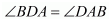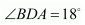Now, in ΔABC , using the property, “exterior angleof a triangle is equal to the sum of its two opposite interior angles”, we get,